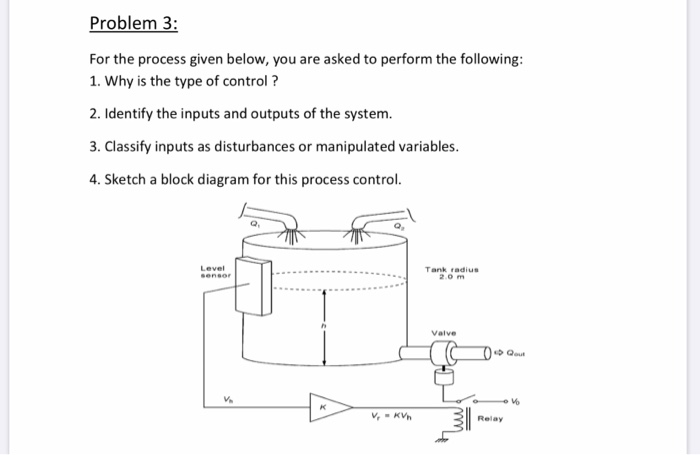# Question Solved1 AnswerProblem 3: For the process given below, you are asked to perform the following: 1. Why is the type of control ? 2. Identify the inputs and outputs of the system. 3. Classify inputs as disturbances or manipulated variables. 4. Sketch a block diagram for this process control. Level sensor Tank radius 20 m Valve Do Que V. V V, KV 31 ww RelayZ5PVSG The Asker · Mechanical EngineeringTranscribed Image Text: Problem 3: For the process given below, you are asked to perform the following: 1. Why is the type of control ? 2. Identify the inputs and outputs of the system. 3. Classify inputs as disturbances or manipulated variables. 4. Sketch a block diagram for this process control. Level sensor Tank radius 20 m Valve Do Que V. V V, KV 31 ww Relay
More
Transcribed Image Text: Problem 3: For the process given below, you are asked to perform the following: 1. Why is the type of control ? 2. Identify the inputs and outputs of the system. 3. Classify inputs as disturbances or manipulated variables. 4. Sketch a block diagram for this process control. Level sensor Tank radius 20 m Valve Do Que V. V V, KV 31 ww Relay